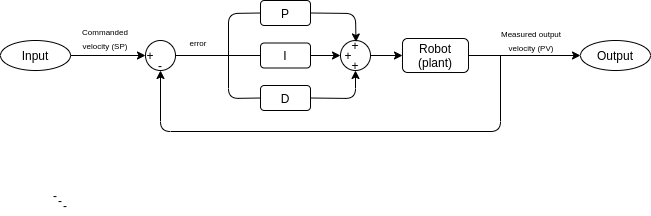# PID Control

One of the most common controllers is a PID-controller, which are currently used to control the actions of the robot. A visual representation of the system is given in Figure 1 below.1. Term P is proportional to the current value of the SP-PV error $e(t)$. For example, if the error is large and positive, the control output will be proportionally large and positive, taking into account the gain factor Kp. Using proportional control alone will result in an error between the set-point and the actual process value because it requires an error to generate the proportional response. If there is no error, there is no corrective response.
For the P action, the error $e(t)$ is multiplied by the gain $K_p$ , so $P = K_p \times e(t)$. For the I action the past errors are summed by an integral and multiplied by a gain called $K_i$ , which makes the second control action $I = K_i \times \int_{0}^{t} e(\tau) d\tau$. The third control action consists of the multiplication of controller gain $K_d$ with the rate of change in error and is described as $D = K_d \times \frac{de(t)}{dt}$. The control actions can be balanced by tuning the controller, which implies changing the gains of the three separate control actions, which regulate the so to say amount of this action.